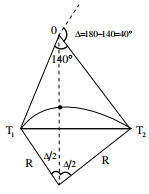# Geomatics Engineering - Online Test

Q1. The survey carried out to delineate natural features, such as hills, rivers, forests and manmade features, such as towns, villages, buildings, roads, transmission lines and canals is classified as
Explaination / Solution:

Topographic survey is carried out to delineate natural features.

Q2. The chainage of the intersection point of two straights is 1585.60 m and the angle of intersection is 140o . If the radius of a circular curve is 600.00 m, the tangent distance (in m) and length of the curve (in m), respectively are
Explaination / Solution:Length of curve, L = (π/180)× ∆×R
= (π/180)×40×600 = 418.88m
Tangent distance, T = R tan(∆/2)
= 600tan(40/2) = 218.38m

Q3. The latitude and departure of a line AB are +78m and −45.1m respectively. The whole circle bearing of the line AB is
Explaination / Solution:Since the latitude of line is positive and departure is negative, the line lies in Fourth quadrant.
l cos θ = 78;  l sin θ = -45.1
tanθ = -0.578
θ = -30o
WCB of AB = 360o-30o = 330o

Q4. As per IS 800: 2007 the cross-section in which extreme fibre can reach the yield stress but cannot develop the plastic moment of resistance due to local buckling is classified as
Explaination / Solution:

As per clause 3.7.2 of IS 800:2007

Q5. Bearing of the given system is shown below:Applying correction due to local attraction, the correct bearing of line BC will be
Explaination / Solution:

The difference between back bearing and fore bearing of line DE
= 258o 30' -78o 30' = 180o
∴ Station D and E are free from local attraction
Fore bearing of line o EA = 212o 30'
∴Correct back bearing of line EA = 216o 30' - 180o = 36o 30'
Error at A = 31o 45' - 36o 30' = -4o 45'; Hence correction at A = +4o 45'
Correct fore bearing of line AB = 126o 45' + 4o 45' = 131o 30'
Correct back bearing of AB = 131o 30 + 180o = 311o 30'
Error at B = -3o 30'; Correction at B = +3o 30'
Hence correct bearing of BC = 45o 15' + 3o 30' = 48o 45'

Q6. The local mean time at a place located in longitude 9040' E when the standard time is 6 hours and 30 minutes and the standard meridian is 8230' E is
Explaination / Solution:
No Explaination.

Q7. A bench mark has been established at the soffit of an ornamental arch at the known elevation of 100.0 m above mean sea level. The back sight used to establish height of instrument is a inverted staffreading of 2.105 m. A forward sight reading with normally held staff of 1.105 m is taken on a recently constructed plinth. The elevation of the plinth is
Explaination / Solution:
No Explaination.

Q8. Curvature correction to a staff reading in a differential leveling survey is
Explaination / Solution:
No Explaination.

Q9. In quadrantal bearing system, bearing of a line varies from
Explaination / Solution:
No Explaination.

Q10. The magnetic bearing of a line AB was N 59o 30' W in the year 1967, when the declination was 4o 10' E. If the present declination is 3o W, the whole circle bearing of the line is
Explaination / Solution:
No Explaination.